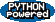PEAK-Rules/Code-Generation UserPreferences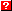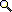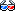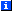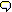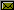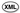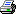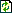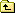The following 624 words could not be found in the dictionary of 50 words (including 50 LocalSpellingWords) and are highlighted below:

# Code Generation from Python Syntax Trees

The peak.rules.codegen module extends peak.util.assembler (from the "BytecodeAssembler" project) with additional AST node types to allow generation of code for simple Python expressions (i.e., those without lambdas, comprehensions, generators, or yields). It also provides "builder" classes that work with the peak.rules.ast_builder module to generate expression ASTs from Python source code, thus creating an end-to-end compiler tool chain, common subexpression caching support, and a state-machine interpreter generator.

This document describes the design (and tests the implementation) of the codegen module. You don't need to read it unless you want to use this module directly in your own programs, or to create specialized add-ons to PEAK-Rules. If you do want to use it directly, keep in mind that it inherits the limitations and restrictions of both peak.util.assembler and peak.rules.ast_builder, so you should consult the documentation for those tools before proceeding.

# AST Generation

To generate an AST from Python code, you need the ast_builder.parse_expr() function, and the codegen.ExprBuilder type:

```>>> from peak.rules.ast_builder import parse_expr
>>> from peak.rules.codegen import ExprBuilder
```

ExprBuilder instances are created using one or more namespaces. The first namespace maps names to arbitrary AST nodes that will be substituted for any matching names found in an expression. The second and remaining namespaces will have their values wrapped in Const nodes, so they can be used for constant-folding. For our examples, we'll define a base namespace containing arguments named "a" through "g":

```>>> from peak.util.assembler import Local
>>> argmap = dict([(name,Local(name)) for name in 'abcdefg'])
>>> builder = ExprBuilder(argmap, locals(), globals(), __builtins__)
```

And, for convenience, we'll save the builder's parse() method as pe:

```>>> pe = builder.parse
```

## Names and Constants

Constants are wrapped in BytecodeAsssembler Const() nodes:

```>>> pe("1")
Const(1)
```

Names found in the first namespace are mapped to whatever value is in the namespace:

```>>> pe("a")
Local('a')
```

Names found in subsequent namespaces get their values wrapped in Const() nodes:

```>>> pe("ExprBuilder")
Const(<...peak.rules.codegen.ExprBuilder...>)

>>> pe("isinstance")
Const(<built-in function isinstance>)
```

And unfound names produce a compile-time error:

```>>> pe("fubar")
Traceback (most recent call last):
...
NameError: fubar
```

## Namespaces and Binding

An ExprBuilder object's bindings attribute is a list of dictionaries, mapping names to the desired outputs:

```>>> builder.bindings
[{}, {'a': Local('a'), 'c': Local('c'), 'b': Local('b'), 'e': Local('e'),
'd': Local('d'), 'g': Local('g'), 'f': Local('f')},
{...}, {...}, {...}]
```

You can add more bindings temporarily with the .push() method, then remove them with .pop():

```>>> builder.push({'q': pe('42')})

>>> builder.Name('q')
Const(42)

>>> builder.pop()
{'q': Const(42)}

>>> builder.Name('q')
Traceback (most recent call last):
...
NameError: q
```

If you omit the argument to .push(), it just adds an empty namespace to the bindings:

```>>> builder.push()
>>> builder.bindings
[{}, {}, {'a': Local('a'), 'c': Local('c'), 'b': Local('b'),
'e': Local('e'), 'd': Local('d'), 'g': Local('g'),
'f': Local('f')}, {...}, {...}, {...}]
```

Which you can then modify using .bind():

```>>> builder.bind({'x': pe('99')})
>>> builder.bindings
[{'x': Const(99)}, {}, {'a': Local('a'), 'c': Local('c'), 'b': Local('b'),
'e': Local('e'), 'd': Local('d'), 'g': Local('g'),
'f': Local('f')}, {...}, {...}, {...}]
```

And finally remove with .pop():

```>>> builder.pop()
{'x': Const(99)}

>>> builder.bindings
[{}, {'a': Local('a'), 'c': Local('c'), 'b': Local('b'), 'e': Local('e'),
'd': Local('d'), 'g': Local('g'), 'f': Local('f')},
{...}, {...}, {...}]
```

## Operators

Unary operators:

```>>> pe("not - + ~ `a`")
Not(Minus(Plus(Invert(Repr(Local('a'))))))
```

Attribute access:

```>>> pe("a.b.c")
Getattr(Getattr(Local('a'), 'b'), 'c')
```

Simple binary operators:

```>>> pe("a+b")
>>> pe("b-a")
Sub(Local('b'), Local('a'))
>>> pe("c*d")
Mul(Local('c'), Local('d'))
>>> pe("c/d")
Div(Local('c'), Local('d'))
>>> pe("c%d")
Mod(Local('c'), Local('d'))
>>> pe("c//d")
FloorDiv(Local('c'), Local('d'))

>>> pe("a**b")
Power(Local('a'), Local('b'))
>>> pe("a<<b")
LeftShift(Local('a'), Local('b'))
>>> pe("a>>b")
RightShift(Local('a'), Local('b'))

>>> pe("a")
Getitem(Local('a'), Const(1))
>>> pe("a")
Getitem(Getitem(Local('a'), Const(1)), Const(2))

>>> pe("a&b&c")
Bitand(Bitand(Local('a'), Local('b')), Local('c'))
>>> pe("a|b|c")
Bitor(Bitor(Local('a'), Local('b')), Local('c'))
>>> pe("a^b^c")
Bitxor(Bitxor(Local('a'), Local('b')), Local('c'))
```

List operators:

```>>> pe("a and b")
And((Local('a'), Local('b')))

>>> pe("a or b")
Or((Local('a'), Local('b')))

>>> pe("a and b and c")
And((Local('a'), Local('b'), Local('c')))

>>> pe("a or b or c")
Or((Local('a'), Local('b'), Local('c')))

>>> pe("[]")
Const([])

>>> pe("[a]")
List((Local('a'),))

>>> pe("[a,b]")
List((Local('a'), Local('b')))

>>> pe("()")
Const(())

>>> pe("a,")
Tuple((Local('a'),))

>>> pe("a,b")
Tuple((Local('a'), Local('b')))
```

Slicing:

```>>> pe("a[:]")
GetSlice(Local('a'), Pass, Pass)

>>> pe("a[1:2]")
GetSlice(Local('a'), Const(1), Const(2))

>>> pe("a[1:]")
GetSlice(Local('a'), Const(1), Pass)

>>> pe("a[:2]")
GetSlice(Local('a'), Pass, Const(2))

>>> pe("a[::]")
Getitem(Local('a'), Const(slice(None, None, None)))

>>> pe("a[1:2:3]")
Getitem(Local('a'), Const(slice(1, 2, 3)))

>>> pe("a[b:c:d]")
Getitem(Local('a'), BuildSlice(Local('b'), Local('c'), Local('d')))
```

Comparisons:

```>>> pe("a>b")
Compare(Local('a'), (('>', Local('b')),))
>>> pe("a>=b")
Compare(Local('a'), (('>=', Local('b')),))
>>> pe("a<b")
Compare(Local('a'), (('<', Local('b')),))
>>> pe("a<=b")
Compare(Local('a'), (('<=', Local('b')),))
>>> pe("a<>b")
Compare(Local('a'), (('!=', Local('b')),))
>>> pe("a!=b")
Compare(Local('a'), (('!=', Local('b')),))
>>> pe("a==b")
Compare(Local('a'), (('==', Local('b')),))
>>> pe("a in b")
Compare(Local('a'), (('in', Local('b')),))
>>> pe("a is b")
Compare(Local('a'), (('is', Local('b')),))
>>> pe("a not in b")
Compare(Local('a'), (('not in', Local('b')),))
>>> pe("a is not b")
Compare(Local('a'), (('is not', Local('b')),))

>>> pe("a>=b>c")
Compare(Local('a'), (('>=', Local('b')), ('>', Local('c'))))
```

Dictionaries:

```>>> pe("{a:b,c:d}")
Dict(((Local('a'), Local('b')), (Local('c'), Local('d'))))
```

Conditional Expressions:

```>>> import sys
>>> if sys.version>='2.5':
...     pe("a if b else c")
... else:
...     print "IfElse(Local('a'), Local('b'), Local('c'))"
IfElse(Local('a'), Local('b'), Local('c'))
```

Calls:

```>>> pe("a()")
Call(Local('a'), (), (), (), (), True)

>>> pe("a(1,2)")
Call(Local('a'), (Const(1), Const(2)), (), (), (), True)

>>> pe("a(1, b=2)")
Call(Local('a'), (Const(1),), ((Const('b'), Const(2)),), (), (), True)

>>> pe("a(*b)")
Call(Local('a'), (), (), Local('b'), (), True)

>>> pe("a(**c)")
Call(Local('a'), (), (), (), Local('c'), True)

>>> pe("a(*b, **c)")
Call(Local('a'), (), (), Local('b'), Local('c'), True)
```

# Bytecode Generation

AST's generated using ExprBuilder can be used directly with BytecodeAssembler Code objects to generate bytecode, complete with constant-folding. Note that the node types not demonstrated below (e.g. And, Or, Compare, Call) are not defined by the codegen module, but instead are imported from peak.util.assembler:

```>>> from peak.rules.codegen import *
>>> from peak.util.assembler import Const, Pass

>>> Minus(1), Plus(2), Not(True), Invert(-1), Repr(4)
(Const(-1), Const(2), Const(False), Const(0), Const('4'))

>>> Add(1,2), Sub(3,2), Mul(4,5), Div(10,2), Mod(7,3), FloorDiv(7,3)
(Const(3), Const(1), Const(20), Const(5), Const(1), Const(2))

>>> Power(2,3), LeftShift(1,4), RightShift(12,2)
(Const(8), Const(16), Const(3))

>>> Getitem(Const([1,2]), 1)
Const(2)

>>> Bitand(3, 1), Bitor(1,2), Bitxor(3,1)
(Const(1), Const(3), Const(2))

>>> Dict([(1,2)])
Const({1: 2})

>>> aList = Const([1,2,3,4])

>>> GetSlice(aList)
Const([1, 2, 3, 4])
>>> GetSlice(aList, 1)
Const([2, 3, 4])
>>> GetSlice(aList, 1, -1)
Const([2, 3])
>>> GetSlice(aList, Pass, -1)
Const([1, 2, 3])

>>> BuildSlice(1, 2, 3)
Const(slice(1, 2, 3))

>>> BuildSlice(1, 2)
Const(slice(1, 2, None))

>>> Tuple([1,2])
Const((1, 2))

>>> List([1,2])
Const([1, 2])

>>> IfElse(1,2,3)
Const(1)

>>> IfElse(1,0,3)
Const(3)
```

## State Machine Interpreter Generation

PEAK-Rules often processes fairly large dispatch trees that would take a long time to generate if translated entirely to bytecode. Plus, they would need to be regenerated every time rules were added to a dispatch tree.

So, instead of generating bytecode that encodes the entire dispatch tree, PEAK-Rules uses a "state machine interpreter" approach. The dispatch tree is represented as a tree of objects. Each node consists of an "action" and an "argument". The generated code is simply an interpreter with inlined bytecode to implement the actions associated with the nodes. To minimize interpretation overhead, actions are encoded in the dispatch tree as jump offsets into the generated bytecode.

Interpreter functions are generated using the SMIGenerator class, instantiated with a function whose calling signature will serve as a template for the interpreter function:

```>>> from peak.rules.codegen import SMIGenerator

>>> def interpreter(input):
...     return input

>>> smig = SMIGenerator(interpreter)
```

To generate the interpreter function, you call the generate() method with a root node: an action/argument tuple:

```>>> exit_node = (0, interpreter)

>>> gfunc = smig.generate(exit_node)
```

The action must either be zero, or a value returned by the action_id() method (described later below). When the generated interpreter encounters action zero, it will treat the argument as a callback. The callback must accept the same number and type of arguments as the interpreter function, and it will be called with the values of the corresponding local variables. The interpreter will invoke the callback, and then exit, returning whatever value or exception was provided by the exit callback:

```>>> gfunc(23)
23
```

Now let's use the same generator, but add some more actions to it. Actions are added using the action_id() method, which takes a code generation target and returns an action ID for use in the interpreter.

The code generation target will execute with no values on the stack, and must finish execution with one value on the stack -- another (action, argument) pair. It can use the generator's ARG attribute to refer to the action argument, and the generator's NEXT_STATE attribute to jump back to the action dispatch loop. A NEXT_STATE jump is automatically generated after each action, so you don't need to include it.

For demonstration and testing, we'll create two new actions: an action that sets the input local variable to its argument, and an action that simply treats the argument as the next state -- a sort of "pass" action. We'll start with the "pass" action:

```>>> pass_id = smig.action_id(smig.ARG)
```

This is about the simplest possible action that meets the requirements of an action: it takes no values on the stack, and puts one value on the stack. In this case, the argument part of the current state.

Now let's create a slightly more complex action, set_input:

```>>> from peak.util.assembler import nodetype

>>> def SetInput(code=None):
...     """Argument is a (value, nextstate) tuple; sets 'input' to value"""
...     if code is None: return ()
...     code(smig.ARG)
...     code.UNPACK_SEQUENCE(2)
...     code.STORE_FAST('input')

>>> SetInput = nodetype()(SetInput)

>>> set_input = smig.action_id(SetInput())
```

This action treats its argument as a (value, nextstate) pair, where value is stored in the input local variable, and nextstate is the next state to proceed to.

By the way, note that Action ID's are cached, so that passing in equivalent code targets will return the same ID each time:

```>>> set_input == smig.action_id(SetInput())
True

>>> pass_id == smig.action_id(smig.ARG)
True

>>> pass_id == smig.action_id(SetInput())
False
```

Whenever you add new actions, you must regenerate the interpreter function in order to be able to use them in the dispatch tree. So we'll regenerate our input function, this time using the set_input action:

```>>> gfunc = smig.generate((set_input, (99, exit_node)))
>>> gfunc(27)
99
```

Now let's create a conditional action and try a more complex tree. This action will proceed to its argument if the input is true, otherwise it will exit immediately:

```>>> input_arg_or_exit = smig.action_id(
...     IfElse(smig.ARG, Local('input'), Const(exit_node))
... )

>>> gfunc = smig.generate(
...     (input_arg_or_exit, (set_input, (True, exit_node)))
... )

>>> gfunc(27)
True

>>> gfunc('')
''
```

By the way, using unrecognized action IDs in a dispatch tree will cause an AssertionError at the point where the action is encountered:

```>>> gfunc = smig.generate(("foo", "bar"))
>>> gfunc(643)
Traceback (most recent call last):
...
AssertionError: Invalid action: foo, bar
```

Finally, note that SMIGenerator objects have a maybe_cache method, that allows you to do subexpression caching as described in the next section:

```>>> smig.maybe_cache
<bound method CSECode.maybe_cache of <...CSECode object...>>
```

Note, however, that the cache lifetime is one full run of the generated interpreter function, so take care when choosing candidate expressions for caching.

## Subexpression Caching

The peak.rules.codegen module includes a common-subexpression caching extension of peak.util.assembler, used to implement "at most once" calculation of any intermediate results during rule evaluation. It works by setting aside a local variable (\$CSECache) to hold a dictionary of temporary values, keyed by strings.

Any time a cached value is needed, the dictionary is checked first. However, the local variable is initially set to None, to avoid creating a dictionary unnecessarily. In this way, only those portions of the dispatch tree that require intermediate expression evaluation will incur the cost of creating or accessing the dictionary.

Note that this caching mechanism is not primarily aimed at improving the performance of the underlying code, although in some cases it might have this effect. It is also not aimed at producing compact code; the code it generates may be considerably larger than the unadorned code would be!

Rather, the goal is to provide the desired semantics (i.e. no duplicated calculations) with better performance than the RuleDispatch package provides for the same operations. In RuleDispatch, expressions are calculated using partial functions and a similar cache dictionary to this one, whereas here the functions are effectively inlined as Python bytecode.

The CSECode class replaces the assembler.Code class:

```>>> from dis import dis

>>> c = CSECode()
>>> a, b = Local('a'), Local('b')

>>> dis(c.code())
```

And the added cache() method takes an expression to cache. If no previous expressions were cached, a preamble is emitted to initialize the cache:

```>>> c.cache(Add(a,b))
>>> dis(c.code())
3 STORE_FAST               0 (\$CSECache)
```

But subsequent cache() calls of course do not repeat the preamble:

```>>> c.cache(Add(a,b))   # deliberate dupe to verify above only happens once
>>> dis(c.code())
3 STORE_FAST               0 (\$CSECache)
```

Generating a cached object results in extra code being added to ensure that the cache variable is initialized and to retrieve the cached value, if present. The resulting code looks complex, but each of the possible code paths are actually fairly short. The cache keys are the string forms of the cached expressions, with an added number to ensure uniqueness:

```>>> c.return_(Add(a,b))
>>> from peak.util.assembler import dump
>>> dump(c.code())
STORE_FAST               0 (\$CSECache)
JUMP_IF_TRUE            L1
POP_TOP
BUILD_MAP                0
DUP_TOP
STORE_FAST               0 (\$CSECache)
L1:     COMPARE_OP               6 (in)
JUMP_IF_FALSE           L2
POP_TOP
BINARY_SUBSCR
JUMP_FORWARD            L3
L2:     POP_TOP
DUP_TOP
STORE_SUBSCR
L3:     RETURN_VALUE
```

While the cache() method marks an expression as definitely cacheable, the maybe_cache() method allows the code object to decide for itself whether the expression should be cached. Specifically, the given expression and all its subexpressions are evaluated against a dummy code object, and its tree structure is examined. Any non-leaf node that appears as a child of two or more parents, or twice or more as a child of the same parent, is considered suitable for caching.

In our first example, the expression (a+b)/c*d is cached, because it's passed to maybe_cache() twice -- once by itself, and once as a child of ((a+b)/c*d) % 3:

```>>> a_plus_b = Add(a,b)
>>> c_times_d = Mul(Local('c'), Local('d'))
>>> abcd = Div(a_plus_b, c_times_d)
>>> m3 = Mod(abcd, 3)

>>> c = CSECode()
>>> c.maybe_cache(abcd)
>>> c.maybe_cache(m3)

>>> c.return_(m3)
>>> dump(c.code())
STORE_FAST               0 (\$CSECache)
JUMP_IF_TRUE            L1
POP_TOP
BUILD_MAP                0
DUP_TOP
STORE_FAST               0 (\$CSECache)
L1:     COMPARE_OP               6 (in)
JUMP_IF_FALSE           L2
POP_TOP
BINARY_SUBSCR
JUMP_FORWARD            L3
L2:     POP_TOP
BINARY_MULTIPLY
BINARY_DIVIDE
DUP_TOP
STORE_SUBSCR
BINARY_MODULO
RETURN_VALUE
```

In the next example, we compute (a+b)*(a+b) after inspecting (a+b)*(b+a) and (b+a)*(a+b) for recurring sub-expressions. Naturally, we detect that (a+b) is used more than once, so it is cached:

```>>> c = CSECode()
>>> ab_2 = Mul(a_plus_b, a_plus_b)
>>> c.maybe_cache(Mul(b_plus_a, a_plus_b))
>>> c.maybe_cache(Mul(a_plus_b, b_plus_a))
>>> c.return_(ab_2)
>>> dump(c.code())
STORE_FAST               0 (\$CSECache)
JUMP_IF_TRUE            L1
POP_TOP
BUILD_MAP                0
DUP_TOP
STORE_FAST               0 (\$CSECache)
L1:     COMPARE_OP               6 (in)
JUMP_IF_FALSE           L2
POP_TOP
BINARY_SUBSCR
JUMP_FORWARD            L3
L2:     POP_TOP
DUP_TOP
STORE_SUBSCR
JUMP_IF_TRUE            L4
POP_TOP
BUILD_MAP                0
DUP_TOP
STORE_FAST               0 (\$CSECache)
L4:     COMPARE_OP               6 (in)
JUMP_IF_FALSE           L5
POP_TOP
BINARY_SUBSCR
JUMP_FORWARD            L6
L5:     POP_TOP
DUP_TOP
STORE_SUBSCR
L6:     BINARY_MULTIPLY
RETURN_VALUE
```

And in this example, we also compute (a+b)*(a+b), but this time only inspecting that one expression for recurrences. We still find the recurrence, because (a+b) occurs more than once under the parent expression:

```>>> c = CSECode()
>>> c.maybe_cache(ab_2)
>>> c.return_(ab_2)
>>> dump(c.code())
STORE_FAST               0 (\$CSECache)
JUMP_IF_TRUE            L1
POP_TOP
BUILD_MAP                0
DUP_TOP
STORE_FAST               0 (\$CSECache)
L1:     COMPARE_OP               6 (in)
JUMP_IF_FALSE           L2
POP_TOP
BINARY_SUBSCR
JUMP_FORWARD            L3
L2:     POP_TOP
DUP_TOP
STORE_SUBSCR
JUMP_IF_TRUE            L4
POP_TOP
BUILD_MAP                0
DUP_TOP
STORE_FAST               0 (\$CSECache)
L4:     COMPARE_OP               6 (in)
JUMP_IF_FALSE           L5
POP_TOP
BINARY_SUBSCR
JUMP_FORWARD            L6
L5:     POP_TOP
DUP_TOP
STORE_SUBSCR
L6:     BINARY_MULTIPLY
RETURN_VALUE
```

Finally, it's important to note that only subexpressions that increase the stack size by exactly 1 are considered for caching:

```>>> from peak.util.assembler import Suite, Code
>>> c = CSECode()
>>> s = Suite([a, b])
>>> ss = Suite([s, s])
>>> c.maybe_cache(ss)
>>> c.return_(ss)
>>> dis(c.code())
12 RETURN_VALUE

>>> c = CSECode()
>>> s = Suite([a, b, Code.POP_TOP, Code.POP_TOP, Code.POP_TOP])
>>> ss = Suite([a, s, a, s])
>>> c.maybe_cache(ss)
>>> c(ss)
>>> dis(c.code())